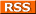Comments Rss
Voronoi Diagrams
http://www.cs.sunysb.edu/~algorith/files/voronoi-diagrams.shtml
SUNY Stony Brook note on Voronoi Diagrams and what they are used for

http://en.wikipedia.org/wiki/Voronoi
WikiPedia: Voronoi (Voronoy) Diagram

I asked a GIS analyst friend of mine, named Larry Braman, to give me his definition of what a voronoi diagram is and its purpose. He gave me this very nice succinct definition. His definition is as follows:

A Voronoi diagram divides the drawing into regions around each point that are shaped so that the borders of the regions are equidistant from the two nearest points. It's kind of the vector equivalent of grid mapping where you're trying to show boundaries of influence from point data.

Not sure where that definition originated, but I like it because its short and simple to understand. Manifold http://www.manifold.net/doc/7x/transform_voronoi_operators.htm has the same definition and has some other interesting tidbits on there site.